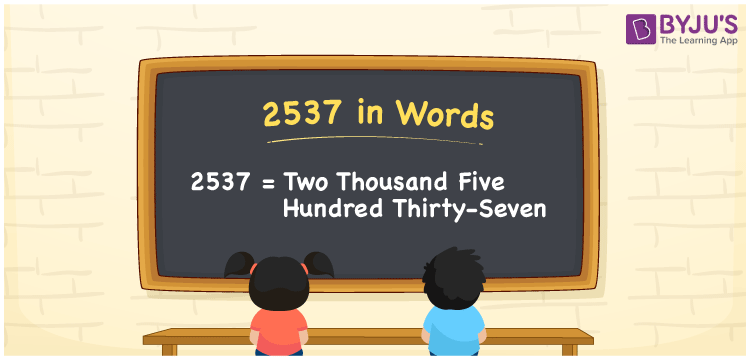# 2537 in Words

We can write 2537 in words as Two thousand five hundred thirty-seven. We can easily convert the given number into word form using a place value chart. If you paid a bill of Rs. 2537 at the stationery shop, then you can say, “I spent Two thousand five hundred thirty-seven rupees for the stationery”. That means we use the word form of cardinal numbers in general discussions. However, in this article, you will learn how to convert the number 2537 into words in detail, along with some interesting facts about 2537.

 2537 in words Two thousand five hundred thirty-seven Two thousand five hundred thirty-seven in Numbers 2537

## 2537 in English Words

Using the English alphabet, we can write numbers in words. So, we can express 2537 in words as “Two thousand five hundred thirty-seven”.## How to Write 2537 in Words?

As we can see, 2537 is a four-digit number so, we need to make a place value chart with four columns such that we can assign a place value for each digit, as shown below.

 Thousands Hundreds Tens Ones 2 5 3 7

Here, ones = 7, tens = 3, hundreds = 5, and thousands = 2.

Let us expand the above numbers.

2 × Thousand + 5 × Hundred + 3 × Ten + 7 × One

= 2 × 1000 + 5 × 100 + 3 × 10 + 7 × 1

= 2000 + 500 + 30 + 7

= Two thousand + Five hundred + Thirty + Seven

= Two thousand five hundred thirty-seven

Therefore, 2537 in words = Two thousand five hundred thirty-seven.

As we know, 2537 is a natural number that precedes 2538 and succeeds 2536.

2537 in words – Two thousand five hundred thirty-seven

Is 2537 an odd number? – Yes

Is 2537 an even number? – No

Is 2537 a prime number? – No

Is 2537 a composite number? – Yes

Is 2537 a perfect square number? – No

Is 2537 a perfect cube number? – No

## Frequently Asked Questions on 2537 in Words

### What number is Two thousand five hundred thirty-seven in words?

The number 2537 is written as Two thousand five hundred thirty-seven in words.

### How to write Rs. 2537 in words on a cheque?

On a cheque, we can write the amount of Rs. 2537 in words as “Two thousand five hundred thirty-seven rupees only”.

### What is the number name of 2537?

The number name of 2537 is Two thousand five hundred thirty-seven.